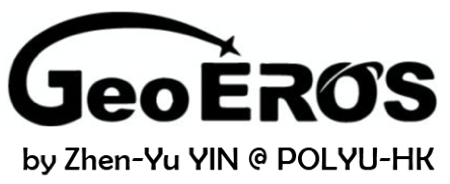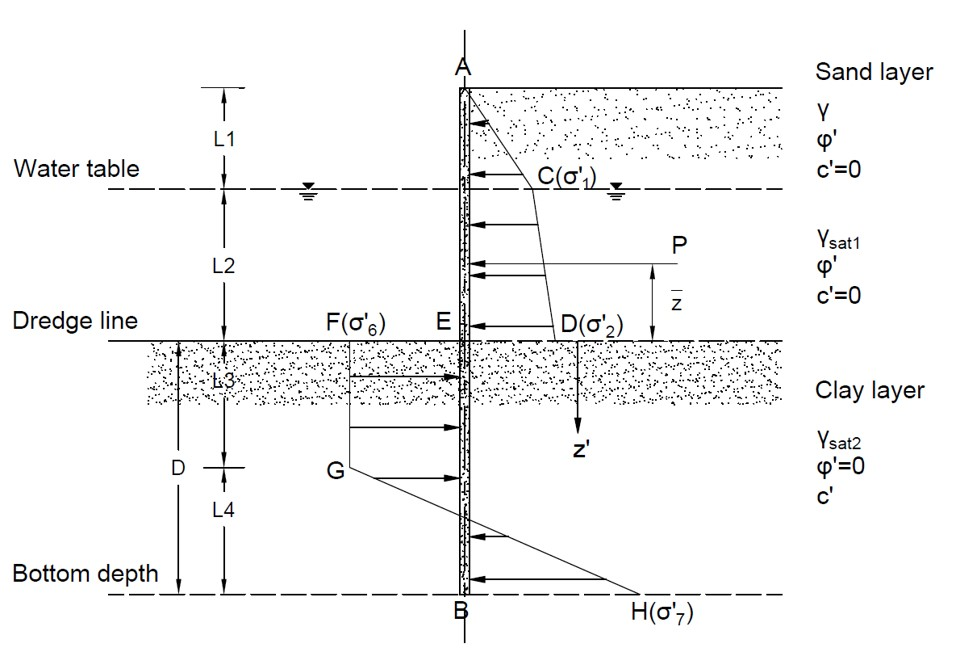# Sheet Pile Wall Tool (Clay)## Geometry of sheet piling penetrating clayey soils## Tips

• This tool is for analysis of sheet piling penetrating clayey soils, as shown in the figure above.
• Two homogenous soil layers are considered in the analysis. The cohesion of sand layer and the friction angle of clay layer are assumed to be zero, and the unit weight of clay layer γsat2 is not used in the calculation.
• The Rankine earth pressure theory is used.
• No surcharge is considered in this case.
• All the input parameters should be positive.
• For "nan", "0" or "inf" displayed in Results, please check your input parameters.

L1 (m)
L2 (m)
γ (kN/m3)
γsat1 (kN/m3)
φ′ (°)
c (kN/m2)
σall (MN/m2)

## Output Results

• Magnitude of pressure diagram
σ′1 (kN/m2) =
σ′2 (kN/m2) =
σ′6 (kN/m2) =
σ′7 (kN/m2) =
• Length of pressure diagram
L3 (m) =
L4 (m) =
• Theoretical embedment depth
Dtheory (m) =
• Center point for pressure of ACDE
z (m) =
• Point for zero shear
z′ (m) =
• Magnitude of maximum moment
Mmax (kN*m/m) =
• Minimum section modulus
S (10-3m3/m) =